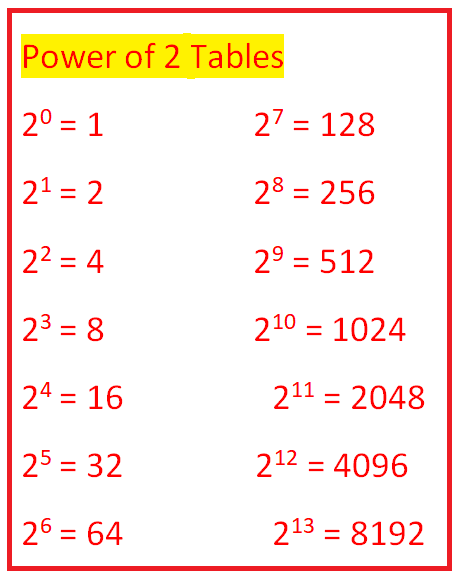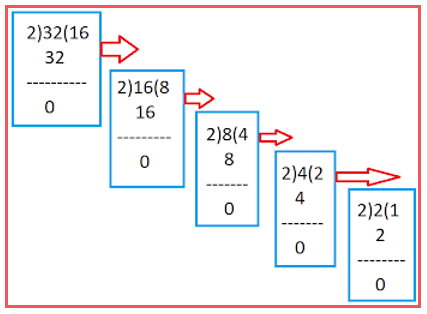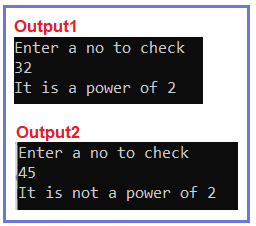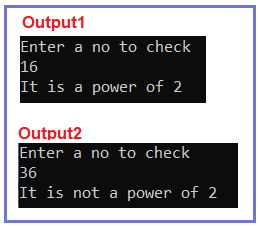# Check Power of 2 or Not in C#

## Check Power of 2 or not in C# with Examples

In this article, I am going to discuss How to Check whether a number is a Power of 2 or not in C# with Examples. Please read our previous article, where we discussed How to Calculate the Perimeter of Square in C#. Given a positive integer Number, The task is to check if the number is a power of 2 or not?

Example1:
Input: 32
Output: It is a power of 2.
Explanation: 2 to the power of 5 is 32(25)

Example2:
Input: 45
Output: It is not the power of 2.

##### Power of 2 Table##### General Logic to be followed.

So, how we are going to solve this problem. Before going to the coding part let’s take a number and example to think of a general way to solve this. For example, 32 is it a power of 2? Yes, but how do we can say that? Because if we break this 32 in terms of 2, we will find exact no of 2’s like 2*2*2*2*2. It means it’s a 2 to the power of 5.

In the same way, we are going to divide the given no by 2 and check if its remainder is equal to 0, then it is divisible by 2. And after each division store the quotient for the next process.As we can see from the above diagram, we are going to divide that number and if its remainder is equal to 0 then store that quotient for the next step and continue the process like that.

One more thing you can observe from the above diagram.is that at last, we are going to get 1 as our quotient if it’s a power of 2. So, on the basis of this, we can use a loop that will run till the quotient will be greater than 1. Now, it’s time for code. Look at the code carefully you will easily understand the code.

##### Algorithm to check whether a number is the power of 2 or not:

Step1: Take input value from user and store into no variable.
Step2: If No is equals to 1 return “It is the power of 2” and stop.
Step3: Declare a variable named remainder.
Step4: Apply remainder operator with no and 2 and store the result into remainder i.e. Remainder = no % 2;
Step5: Check if the remainder is not equal to 0. Then immediately break the loop.
Step6: Apply division operator with no and 2 i.e. no = no /2;
Step7: Repeat Steps 4, 5, and 6 until no is greater than 1.
Step8: Check if no is equals to 1 Print “Power of 2” else print “Power of not 2”.

##### Example: C# Program to Check Power of 2 or not

The following C# Program will check whether a given number is a power of 2 or not.

```using System;
namespace DotNetTutorials
{
public class PowerOf2
{
static void Main(string[] args)
{
try
{
int no;
int remainder;
Console.WriteLine("Enter a no to check");

if (no == 1)
Console.Write("It is power of 2.");
else
{
while (no > 1)
{
remainder = no % 2;
if (remainder != 0)
break;
no /= 2;
}
if (no == 1)
Console.WriteLine("It is a power of 2");
else
Console.WriteLine("It is not a power of 2");
}
}
catch (Exception ex)
{
Console.WriteLine(ex.Message);
}

}
}
}
```
###### Output:##### C# Code (Using function)

In the below C# example, we have created one method to check whether a given number is the power of 2 or not.

```using System;
namespace DotNetTutorials
{
public class PowerOf2
{
static void Main(string[] args)
{
try
{
int no;
Console.WriteLine("Enter a no to check");
PowerOf2 pr = new PowerOf2();
bool check = pr.IsPowerOf2(no);
if (check)
Console.WriteLine("It is a power of 2");
else
Console.WriteLine("It is not a power of 2");
}
catch (Exception ex)
{
Console.WriteLine(ex.Message);
}
}

public bool IsPowerOf2(int no)
{
if (no == 1)
return true;
int remainder;
while (no > 1)
{
remainder = no % 2;
if (remainder != 0)
break;
no /= 2;
}
if (no == 1)
return true;
else
return false;
}
}
}
```
###### Output:In the next article, I am going to discuss How to count the number of 1 bit in a given number in C# with Examples. Here, in this article, I try to explain How to Check if a given number is the power of 2 or not in C# with Examples and I hope you enjoy this How to Check if a given number is the power of 2 or not in C# article.

### 1 thought on “Check Power of 2 or Not in C#”

1.using System;

namespace LogicalPrograms
{
public class Program
{
static void Main(string[] args)
{
int number = 8;
int result = 1;

int i;
for (i = 1; i number)
{
Console.WriteLine(“not a power of 2”);
}